# EOPatch visualization

This tutorial showcases EOPatch visualization functionalities. We are going to load and visualize features from TestEOPatch.

:

%matplotlib inline

import os

from eolearn.core import EOPatch, FeatureType

EOPATCH_PATH = os.path.join("..", "..", "example_data", "TestEOPatch")

eopatch

:

EOPatch(
data={
BANDS-S2-L1C: numpy.ndarray(shape=(68, 101, 100, 13), dtype=float32)
CLP: numpy.ndarray(shape=(68, 101, 100, 1), dtype=float32)
CLP_MULTI: numpy.ndarray(shape=(68, 101, 100, 1), dtype=float32)
CLP_S2C: numpy.ndarray(shape=(68, 101, 100, 1), dtype=float32)
NDVI: numpy.ndarray(shape=(68, 101, 100, 1), dtype=float32)
REFERENCE_SCENES: numpy.ndarray(shape=(5, 101, 100, 13), dtype=float32)
}
CLM: numpy.ndarray(shape=(68, 101, 100, 1), dtype=uint8)
CLM_INTERSSIM: numpy.ndarray(shape=(68, 101, 100, 1), dtype=bool)
CLM_MULTI: numpy.ndarray(shape=(68, 101, 100, 1), dtype=bool)
CLM_S2C: numpy.ndarray(shape=(68, 101, 100, 1), dtype=bool)
IS_DATA: numpy.ndarray(shape=(68, 101, 100, 1), dtype=uint8)
IS_VALID: numpy.ndarray(shape=(68, 101, 100, 1), dtype=bool)
}
scalar={
CLOUD_COVERAGE: numpy.ndarray(shape=(68, 1), dtype=float16)
}
label={
IS_CLOUDLESS: numpy.ndarray(shape=(68, 1), dtype=bool)
RANDOM_DIGIT: numpy.ndarray(shape=(68, 2), dtype=int8)
}
vector={
CLM_VECTOR: geopandas.GeoDataFrame(columns=['TIMESTAMP', 'VALUE', 'geometry'], length=55, crs=EPSG:32633)
}
data_timeless={
DEM: numpy.ndarray(shape=(101, 100, 1), dtype=float32)
MAX_NDVI: numpy.ndarray(shape=(101, 100, 1), dtype=float64)
}
LULC: numpy.ndarray(shape=(101, 100, 1), dtype=uint16)
RANDOM_UINT8: numpy.ndarray(shape=(101, 100, 13), dtype=uint8)
VALID_COUNT: numpy.ndarray(shape=(101, 100, 1), dtype=int64)
}
scalar_timeless={
LULC_PERCENTAGE: numpy.ndarray(shape=(6,), dtype=float64)
}
label_timeless={
LULC_COUNTS: numpy.ndarray(shape=(6,), dtype=int32)
}
vector_timeless={
LULC: geopandas.GeoDataFrame(columns=['index', 'RABA_ID', 'AREA', 'DATE', 'LULC_ID', 'LULC_NAME', 'geometry'], length=88, crs=EPSG:32633)
}
meta_info={
maxcc: 0.8
service_type: 'wcs'
size_x: '10m'
size_y: '10m'
}
bbox=BBox(((465181.0522318204, 5079244.8912012065), (466180.53145382757, 5080254.63349641)), crs=CRS('32633'))
timestamps=[datetime.datetime(2015, 7, 11, 10, 0, 8), ..., datetime.datetime(2017, 12, 22, 10, 4, 15)], length=68
)


## Basics

All visualizations can be done simply by calling EOPatch.plot method, however calling this method still requires that eo-learn-visualization subpackage is installed.

Plotting a simple timeless single-channel feature produces a single-image plot. Plotting method always returns a 2D grid of AxesSubplot objects which can be further customized before a plot is produced.

:

axes_grid = eopatch.plot((FeatureType.DATA_TIMELESS, "MAX_NDVI"))

print("A 2D grid:", axes_grid)

figure = axes_grid.figure
figure.set_dpi(40)

A 2D grid: [[<AxesSubplot:>]]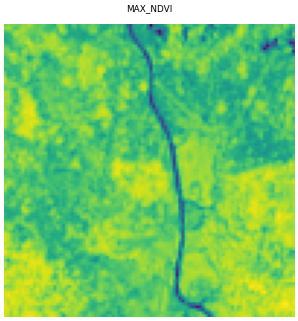## Plotting filters and configurations

Let’s plot a feature containing Sentinel-2 bands. It will create a grid of subplots where every row contains images for the same timestamp and every column contains images from the same channel.

Because plotting a grid of 68 x 13 images would take too much time and memory we’ll use filters to plot only some timestamps and channels. Filtering parameters support either a slice object or a list of indices to keep. Additionally, we can write the names of channels to be written next to each image.

:

eopatch.plot(
(FeatureType.DATA, "BANDS-S2-L1C"), times=slice(3, 6), channels=[2, 5, 10], channel_names=["B03", "B06", "B10"]
);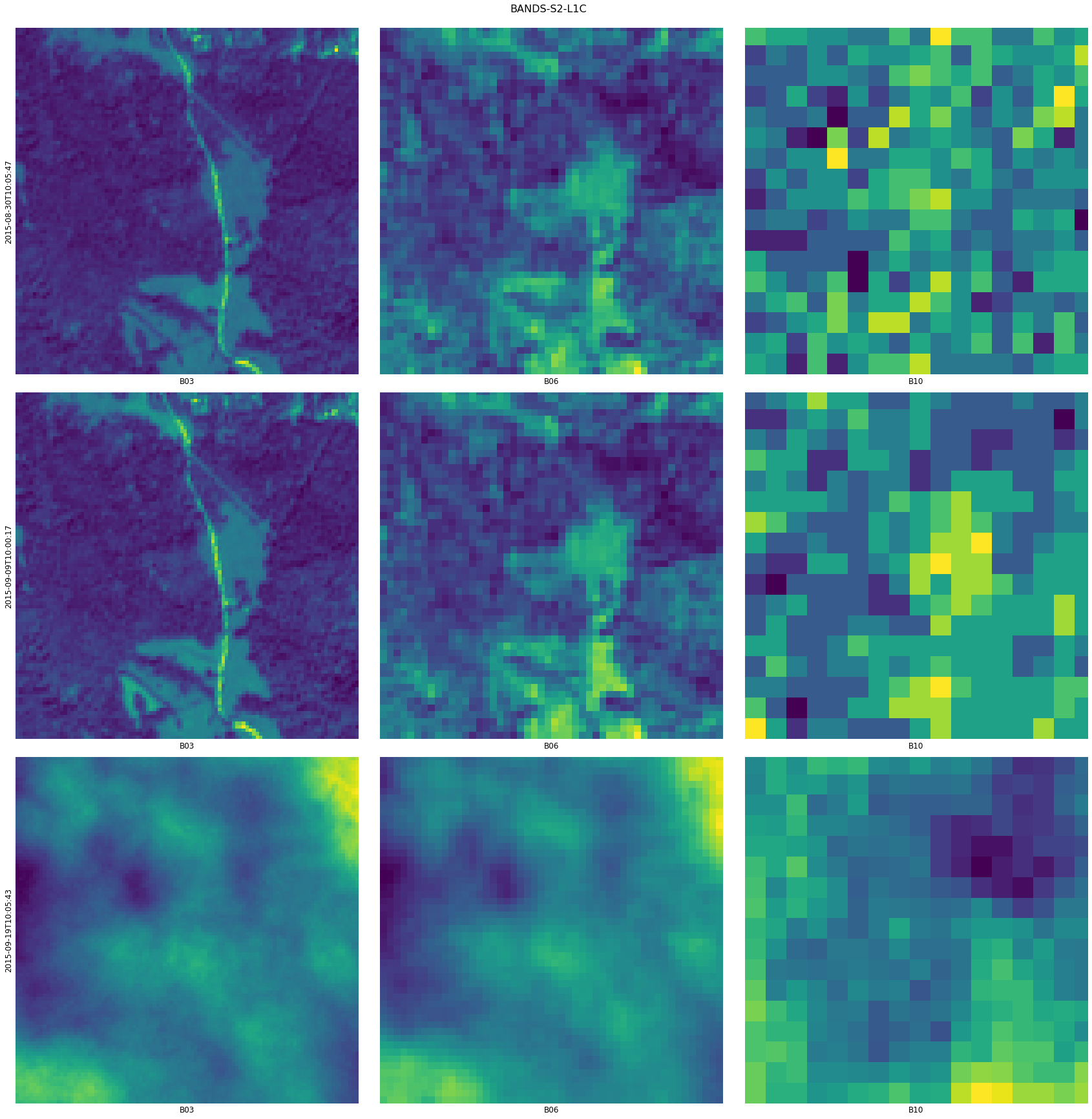We can also select any 3 channels and plot RGB images.

:

eopatch.plot((FeatureType.DATA, "BANDS-S2-L1C"), times=[4, 10, 20], rgb=[3, 2, 1], channel_names=["TRUE COLOR"]);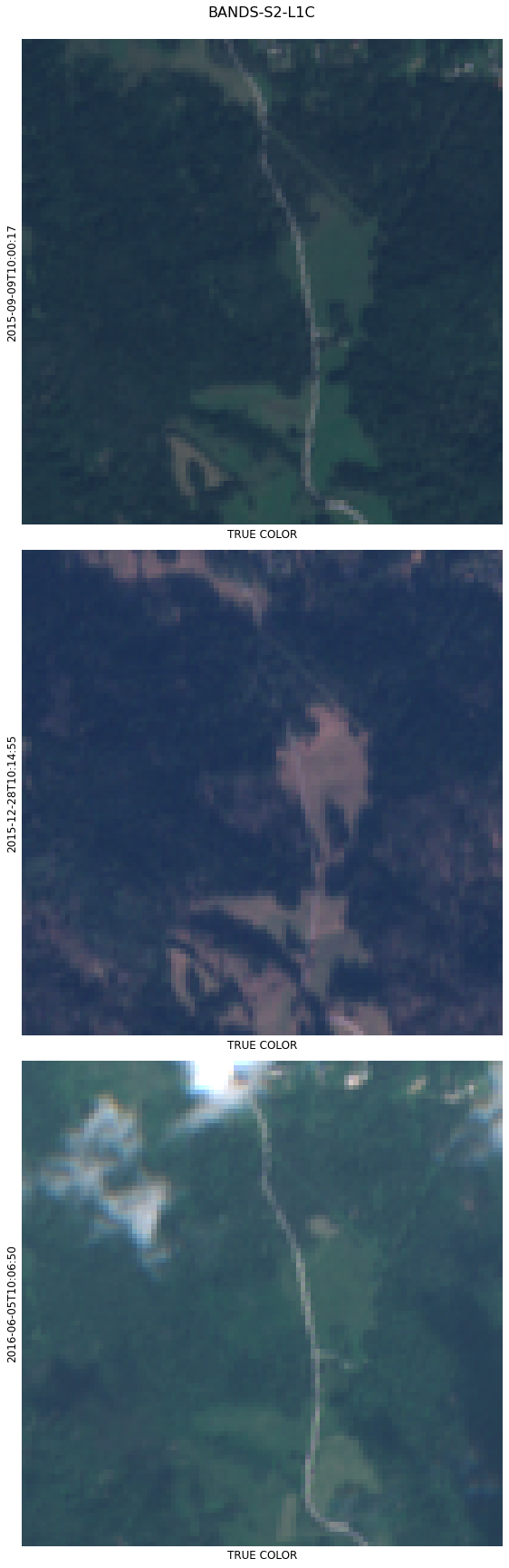Plotting also supports plenty of advanced low-level configuration options. Those can be configured with a PlotConfig object.

:

from eolearn.visualization import PlotConfig

config = PlotConfig(subplot_width=5, subplot_height=5, rgb_factor=1, show_title=False)

eopatch.plot(
(FeatureType.DATA, "BANDS-S2-L1C"), times=[4, 10, 20], rgb=[7, 3, 2], channel_names=["FALSE COLOR"], config=config
);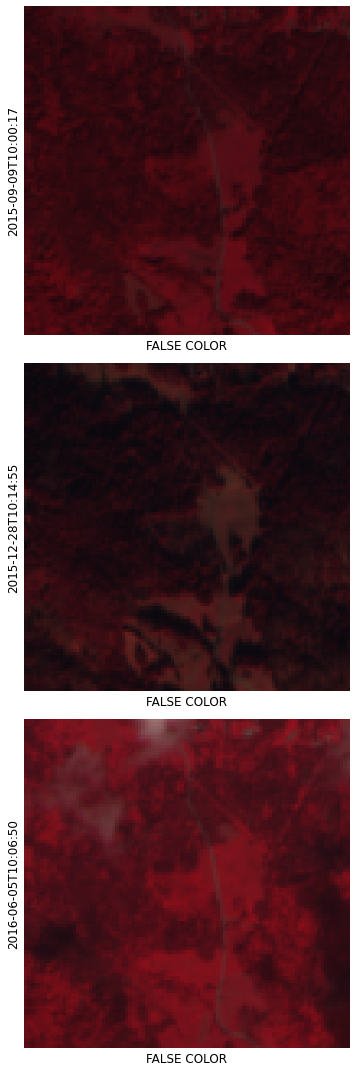## Types of plots

Next, let’s check what kind of plots other feature types produce. Non-spatial temporal raster features are plotted as time series of values.

:

eopatch.plot(
(FeatureType.SCALAR, "CLOUD_COVERAGE"),
channel_names=["Cloud coverage percentage"],
config=PlotConfig(subplot_width=16),
);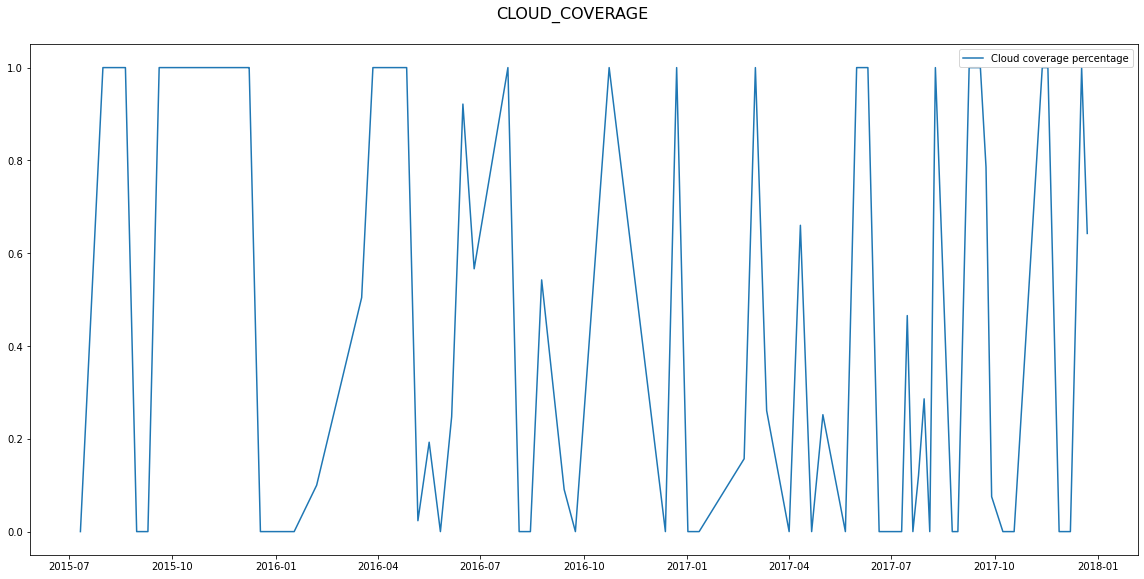Timeless non-spatial raster features produce histogram plots.

:

eopatch.plot(
(FeatureType.LABEL_TIMELESS, "LULC_COUNTS"),
channel_names=["Cultivated Land", "Forest", "Grassland", "Water", "Artificial Surface", "Bareland"],
);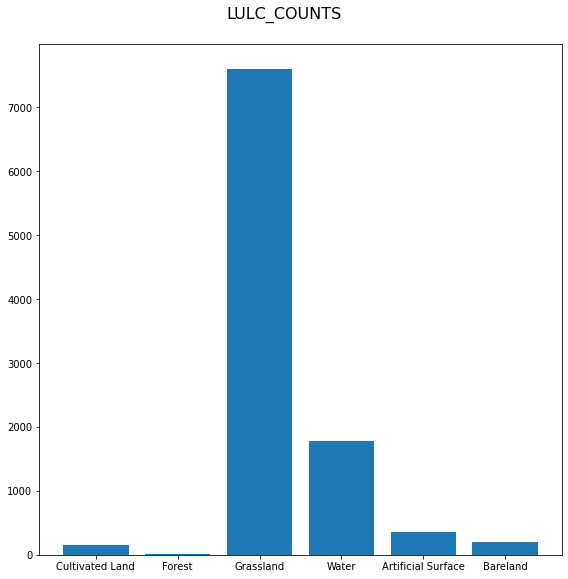Vector features are plotted together with an EOPatch bounding box.

:

eopatch.plot((FeatureType.VECTOR, "CLM_VECTOR"), times=slice(6, 9));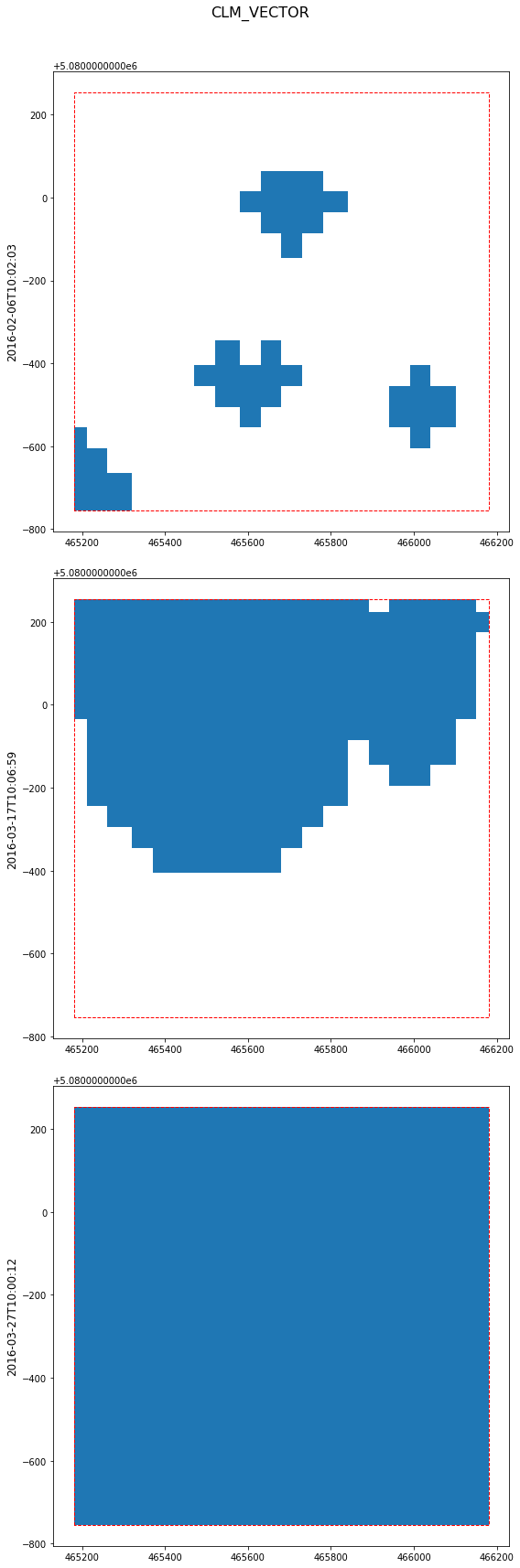Additionally we can plot a bounding box feature on its own.

:

eopatch.plot(FeatureType.BBOX);